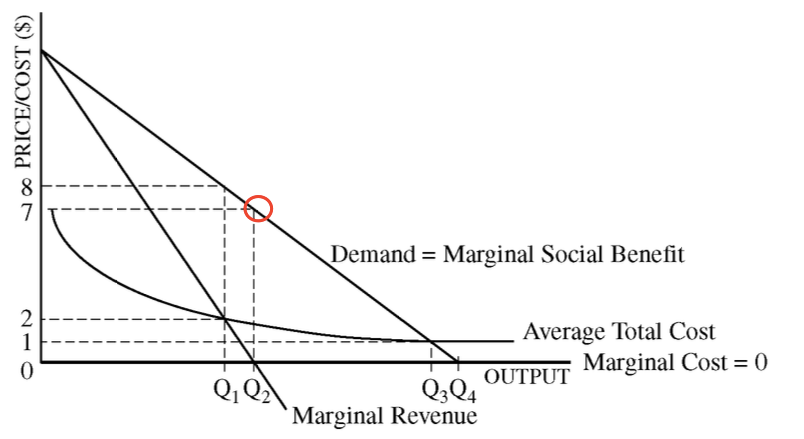Question 1 (a)

• Profit-maximizing pointQuestion 1 (c)

• Economic profit = Total revenue - Explicit costs - Implicit costs.

• If the firm earns zero economic profit, the accounting profit is positive.

Question 1 (f)

• Suppose the long-run average total cost is strictly downward sloping. Would it be efficient to build a second bridge? Explain.

• One point is earned for stating that building a second bridge would be inefficient and for explaining that, because there are economies of scale, building a second bridge would raise the average total cost.

Question 2 (b)

• The law of diminishing returns

• As more and more units of a variable input are added to a fixed input, the output increases at a decreasing rate.• Diminishing returns occur because of the overuse of the fixed input.

Question 3 (a)

• Elasticity and tax